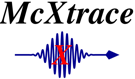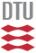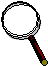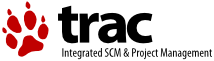McXtrace: Source_flat Component

# The `Source_flat` Component

A flat rectangular or circular surface emitting x-rays

## Identification

• Author:Erik Knudsen
• Origin: Risoe
• Date: September 25, 2009
• Version: \$Revision\$

## Description

```A circular or rectangular xray source. Spectrum may be either gaussian or uniform around a central wavelength/energy
or read from a datafile. Xrays are considered emitted uniformly into 4pi, but a square target retricts the beam to
that window and scales the beam intensity accordingly.
If an input spectrum datafile (spectrum_file) is not specified, the beam is restricted to emit photons between E0+-dE keV, or lambda0+-dlambda AA, whichever is given.
The input spectrum file should be formatted such that x-ray energy/wavelength is in the first column and the intensity in the second. Any preceding
lines starting with # are considered part of the file header. If a datafile is given, a nonzero E0 value indicates that is is parametrized by energy (in keV)
as opposed to wavelength (in AA). Wavelength is the default.
Flux is set in the unit photons/s

```

## Input parameters

Parameters in boldface are required; the others are optional.
Name Unit Description Default
spectrum_file NULL
radius m Radius of circle in (x,y,0) plane where x-rays are generated. 0
yheight m Height of rectangle in (x,y,0) plane where x-rays are generated. 0
xwidth m Width of rectangle in (x,y,0) plane where x-rays are generated. Overrides xmin and xmax. 0
xmin m Lower bound of x-interval where photons are generated. 0
xmax m upper bound of x-interval where photons are generated. 0
dist m Distance to target along z axis. 0
focus_xw m Width of target .045
focus_yh m Height of target .12
E0 keV Mean energy of xrays. 0
dE keV Energy half spread of x-rays (flat or gaussian sigma). 0
lambda0 AA Mean wavelength of x-rays. 0
dlambda AA Wavelength half spread of x-rays. 0
flux pht/s total flux radiated from the source 0
gauss 1 Gaussian (1) or Flat (0) energy/wavelength distribution 0
randomphase If nonzero, the phase of the emotted photon is random, i.e. source is fully incoherent. otherwise the value of phase is used. 1
phase Set phase to something given. 0

## Output parameters

Name Unit Description Default
prms
square
pmul
srcArea